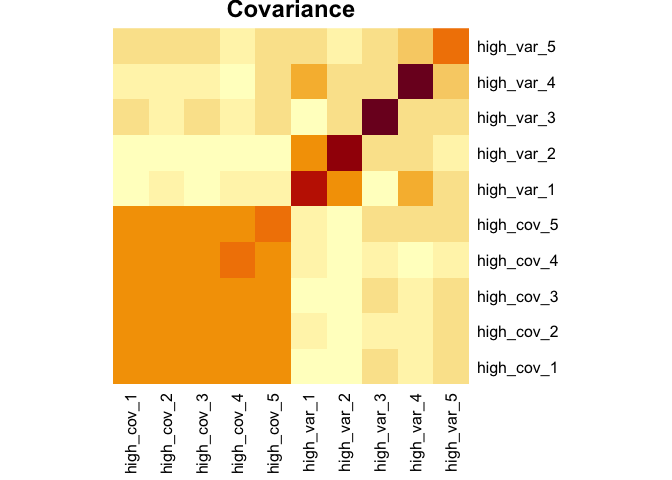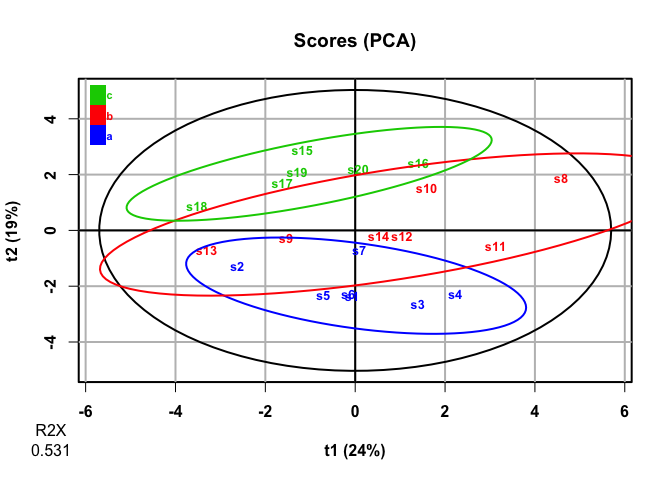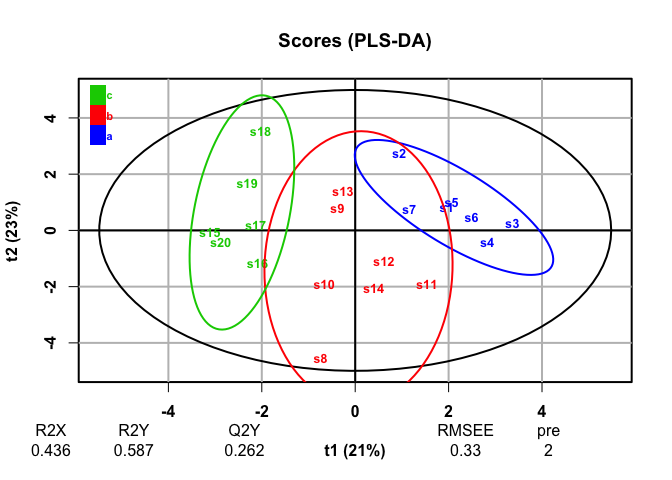# holodeck: A Tidy Interface For Simulating Multivariate Data

`holodeck` allows quick and simple creation of simulated multivariate data with variables that co-vary or discriminate between levels of a categorical variable. The resulting simulated multivariate dataframes are useful for testing the performance of multivariate statistical techniques under different scenarios, power analysis, or just doing a sanity check when trying out a new multivariate method.

## Installation

From CRAN:

``install.packages("holodeck)``

Development version from r-universe:

``install.packages('holodeck', repos = c('https://aariq.r-universe.dev', 'https://cloud.r-project.org'))``

`holodeck` is built to work with `dplyr` functions, including `group_by()` and the pipe (`%>%`). `purrr` is helpful for iterating simulated data. For these examples I’ll use `ropls` for PCA and PLS-DA.

``````library(holodeck)
library(dplyr)
library(tibble)
library(purrr)
library(ropls)``````

## Example 1: Investigating PCA and PLS-DA

Let’s say we want to learn more about how principal component analysis (PCA) works. Specifically, what matters more in terms of creating a principal component—variance or covariance of variables? To this end, you might create a dataframe with a few variables with high covariance and low variance and another set of variables with low covariance and high variance

### Generate data

``````set.seed(925)
df1 <-
sim_covar(n_obs = 20, n_vars = 5, cov = 0.9, var = 1, name = "high_cov") %>%
sim_covar(n_vars = 5, cov = 0.1, var = 2, name = "high_var") ``````

Explore covariance structure visually. The diagonal is variance.

``````df1 %>%
cov() %>%
heatmap(Rowv = NA, Colv = NA, symm = TRUE, margins = c(6,6), main = "Covariance")``````Now let’s make this dataset a little more complex. We can add a factor variable, some variables that discriminate between the levels of that factor, and add some missing values.

``````set.seed(501)
df2 <-
df1 %>%
sim_cat(n_groups = 3, name = "factor") %>%
group_by(factor) %>%
sim_discr(n_vars = 5, var = 1, cov = 0, group_means = c(-1.3, 0, 1.3), name = "discr") %>%
sim_discr(n_vars = 5, var = 1, cov = 0, group_means = c(0, 0.5, 1), name = "discr2") %>%
sim_missing(prop = 0.1) %>%
ungroup()
df2
#> # A tibble: 20 × 21
#>    factor high_cov_1 high_cov_2 high_cov_3 high_cov_4 high_cov_5 high_var_1
#>    <chr>       <dbl>      <dbl>      <dbl>      <dbl>      <dbl>      <dbl>
#>  1 a           0.472   -0.362       0.253      0.281      NA        -0.0873
#>  2 a          -1.50    -1.65       -1.47      -1.93       NA        NA
#>  3 a           1.13    NA          NA          1.41        0.345     0.871
#>  4 a           0.982    0.740       1.16       1.14        0.866    NA
#>  5 a          -0.773   NA          NA         -1.21       -1.25     -1.53
#>  6 a           0.302    0.130      -0.309      0.0725      0.725     0.890
#>  7 a          -0.117    0.00163     0.0596    -0.542      -0.269    NA
#>  8 b           2.16     2.47        1.38       1.62        1.62     -2.43
#>  9 b          NA       -0.509      -0.529     -0.842      -1.04      1.25
#> 10 b           0.609    0.195       0.720      0.930       0.595    -0.562
#> 11 b           1.81     1.15        1.43       1.09        1.39     -0.934
#> 12 b           0.954    0.234       0.247      0.248       0.751     1.95
#> 13 b          -1.03    NA          -1.70      -1.27       -1.64      0.670
#> 14 b          NA        0.380       0.177     NA           0.550     2.68
#> 15 c          -0.214   -0.390      -0.476     -0.878      -0.328     0.665
#> 16 c           0.827    0.556       0.620      0.491       0.814    -0.0121
#> 17 c          -0.399   -0.862      -0.385     -0.935      -0.802    NA
#> 18 c          -1.09    -1.32       -0.720     -1.88       -1.76     -2.05
#> 19 c          -0.181   -0.155      -0.774      0.0395     -0.770     1.81
#> 20 c           0.882   NA           0.758      1.24       NA         1.11
#> # ℹ 14 more variables: high_var_2 <dbl>, high_var_3 <dbl>, high_var_4 <dbl>,
#> #   high_var_5 <dbl>, discr_1 <dbl>, discr_2 <dbl>, discr_3 <dbl>,
#> #   discr_4 <dbl>, discr_5 <dbl>, discr2_1 <dbl>, discr2_2 <dbl>,
#> #   discr2_3 <dbl>, discr2_4 <dbl>, discr2_5 <dbl>``````

### PCA

``````pca <- opls(select(df2, -factor), fig.pdfC = "none", info.txtC = "none")

plot(pca, parAsColFcVn = df2\$factor, typeVc = "x-score")````````````
as_tibble(rownames = "variable") %>%
arrange(desc(abs(p1)))
#> # A tibble: 20 × 4
#>    variable         p1      p2       p3
#>    <chr>         <dbl>   <dbl>    <dbl>
#>  1 high_cov_2  0.415    0.0534  0.0137
#>  2 high_cov_1  0.407    0.0383  0.0208
#>  3 high_cov_5  0.401    0.0163  0.104
#>  4 high_cov_3  0.400    0.0301 -0.0837
#>  5 high_cov_4  0.387    0.0218  0.00556
#>  6 discr_5    -0.224    0.262  -0.136
#>  7 high_var_2 -0.195    0.0848  0.240
#>  8 discr2_1    0.167    0.396  -0.167
#>  9 discr_2    -0.163    0.322  -0.202
#> 10 high_var_5  0.115   -0.132   0.261
#> 11 discr2_5    0.0967   0.267   0.114
#> 12 high_var_1 -0.0930  -0.0102  0.457
#> 13 discr2_4    0.0834   0.308   0.0924
#> 14 discr_3    -0.0627   0.376  -0.0152
#> 15 discr2_2   -0.0412   0.138   0.539
#> 16 discr_1    -0.0407   0.319   0.0471
#> 17 discr2_3   -0.0394   0.176  -0.358
#> 18 discr_4     0.0363   0.400   0.144
#> 19 high_var_3 -0.0101  -0.0629  0.0483
#> 20 high_var_4 -0.00471  0.131   0.308``````

It looks like PCA mostly picks up on the variables with high covariance, not the variables that discriminate among levels of `factor`. This makes sense, as PCA is an unsupervised analysis.

### PLS-DA

``````plsda <- opls(select(df2, -factor), df2\$factor, predI = 2, permI = 10, fig.pdfC = "none", info.txtC = "none")

plot(plsda, typeVc = "x-score")````````````
getVipVn(plsda) %>%
tibble::enframe(name = "variable", value = "VIP") %>%
arrange(desc(VIP))
#> # A tibble: 20 × 2
#>    variable      VIP
#>    <chr>       <dbl>
#>  1 discr_4    1.54
#>  2 discr_1    1.48
#>  3 discr_2    1.47
#>  4 discr_5    1.44
#>  5 discr_3    1.42
#>  6 discr2_1   1.31
#>  7 discr2_4   1.09
#>  8 high_cov_2 1.08
#>  9 discr2_3   0.996
#> 10 high_cov_1 0.944
#> 11 discr2_2   0.884
#> 12 high_cov_5 0.790
#> 13 discr2_5   0.650
#> 14 high_var_5 0.639
#> 15 high_var_2 0.582
#> 16 high_cov_4 0.530
#> 17 high_cov_3 0.423
#> 18 high_var_4 0.358
#> 19 high_var_1 0.200
#> 20 high_var_3 0.0860``````

PLS-DA, a supervised analysis, finds discrimination among groups and finds that the discriminating variables we generated are most responsible for those differences.